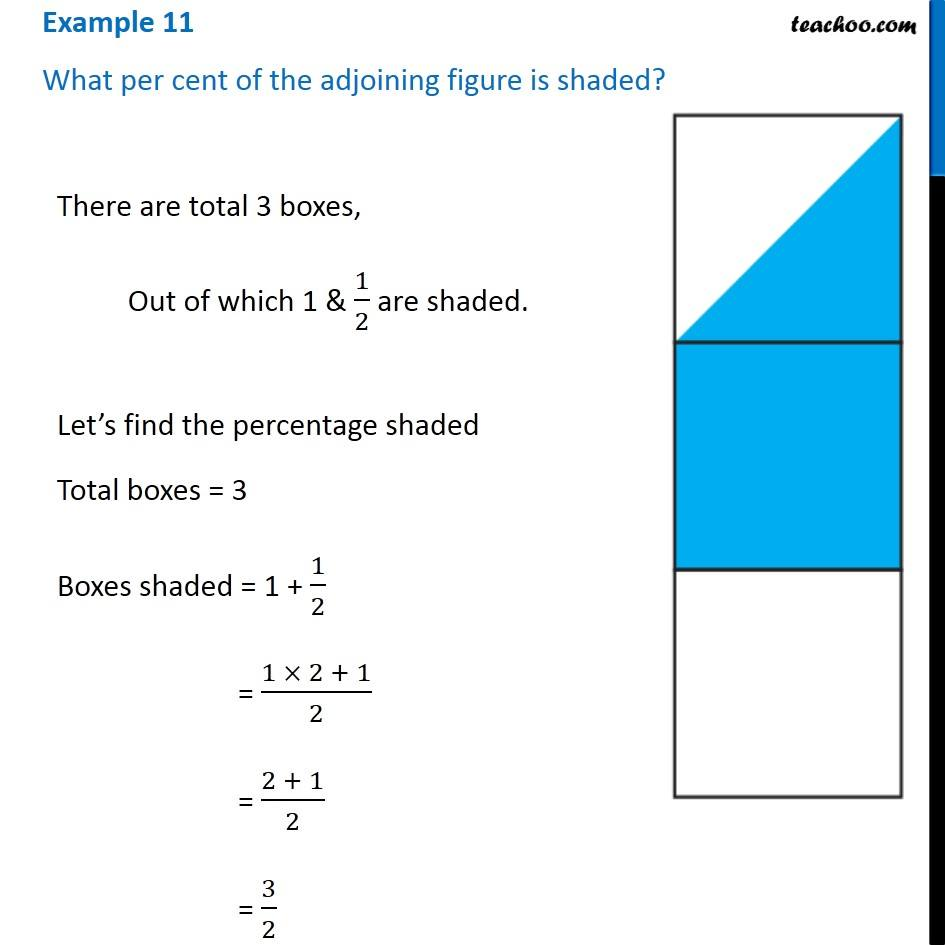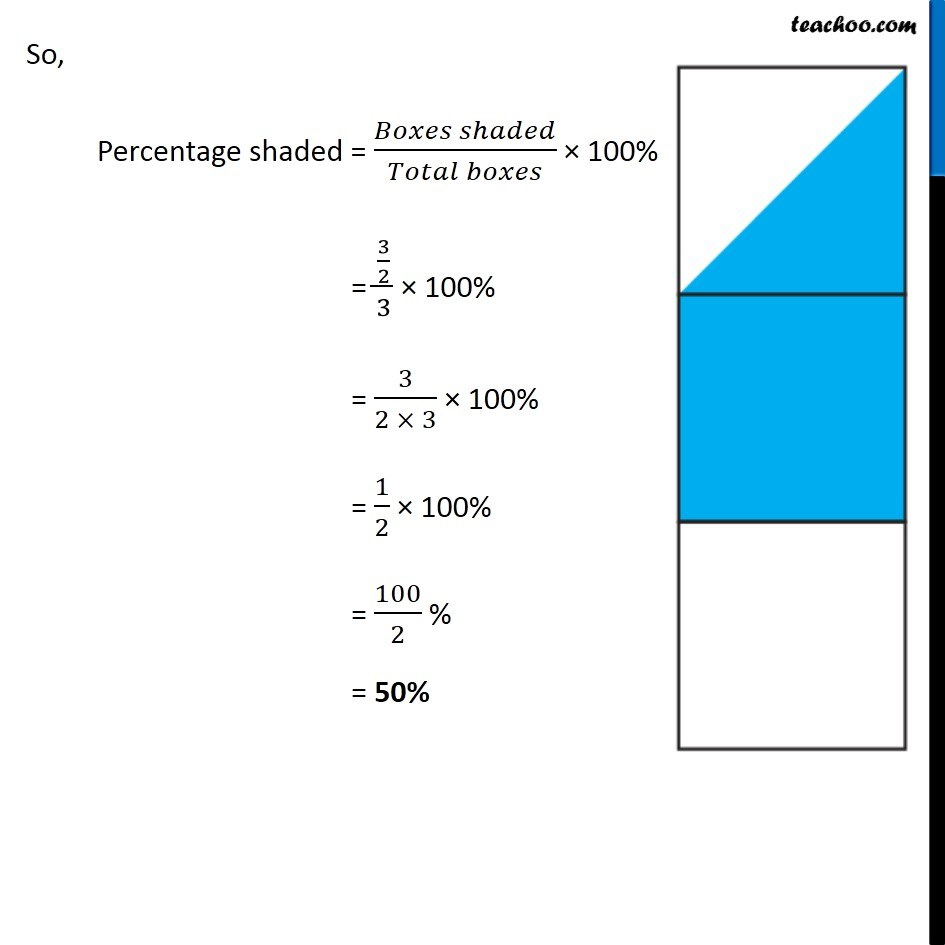Subscribe to our Youtube Channel - https://you.tube/teachoo

1. Chapter 8 Class 7 Comparing Quantities
2. Concept wise
3. Converting fraction to percent

Transcript

Example 11 What per cent of the adjoining figure is shaded? There are total 3 boxes, Out of which 1 & 1/2 are shaded. Let’s find the percentage shaded Total boxes = 3 Boxes shaded = 1 + 1/2 = (1 × 2 + 1)/2 = (2 + 1)/2 = 3/2 So, Percentage shaded = (𝐵𝑜𝑥𝑒𝑠 𝑠ℎ𝑎𝑑𝑒𝑑)/(𝑇𝑜𝑡𝑎𝑙 𝑏𝑜𝑥𝑒𝑠) × 100% = (3/2)/3 × 100% = 3/(2 × 3) × 100% = 1/2 × 100% = 100/2 % = 50%

Converting fraction to percent

About the AuthorDavneet Singh
Davneet Singh is a graduate from Indian Institute of Technology, Kanpur. He has been teaching from the past 9 years. He provides courses for Maths and Science at Teachoo.